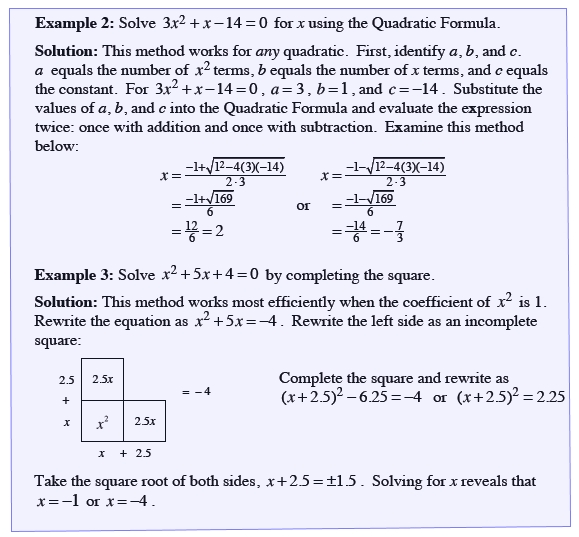Home > CCA > Chapter 9 > Lesson 9.4.3 > Problem9-117

9-117.

Solve the quadratic equation below twice, once using the Quadratic Formula and once by completing the square. Which was easier? Homework Help ✎

$x^2−10x+21=−4$

Follow the steps in the Math Notes box.